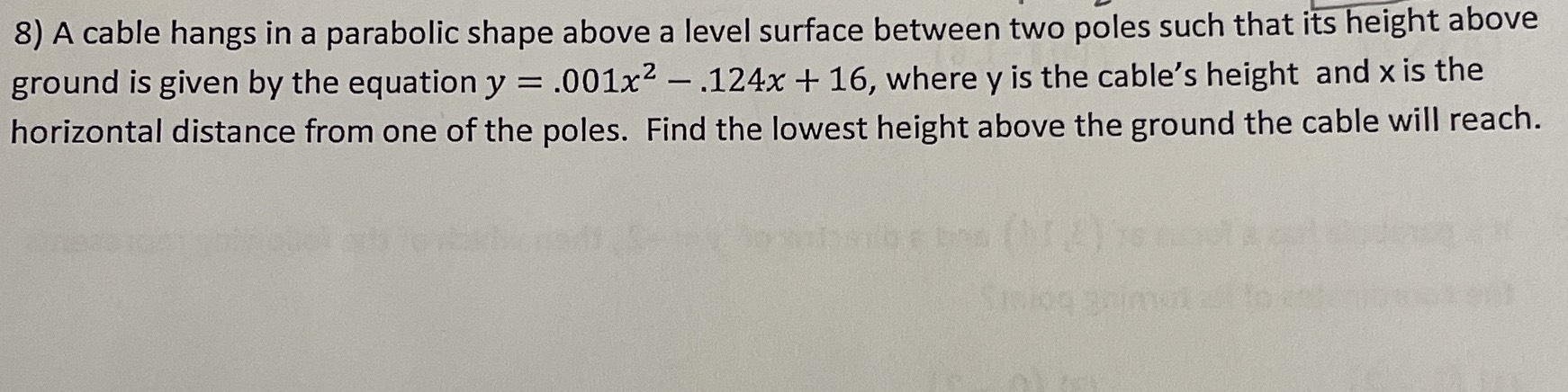### ¿Todavía tienes preguntas de matemáticas?

Pregunte a nuestros tutores expertos
Algebra
PreguntaA cable hangs in a parabolic shape above a level surface between two poles such that its height above ground is given by the equation $$y = .001 x ^ { 2 } - .124 x + 16 ,$$ where $$y$$ is the cable's height and $$x$$ is the horizontal distance from one of the poles. Find the lowest height above the ground the cable will reach.